# Introduction to Trigonometry Notes

In this page we will explain the topics for the chapter 8 of Introduction to Trigonometry Class 10 Maths.We have given quality Introduction to Trigonometry Class 10 Notes to explain various things so that students can benefits from it and learn maths in a fun and easy manner, Hope you like them and do not forget to like , social share and comment at the end of the page.
Table of Content

## What is Trigonometry

• Trigomometry from Greek trigõnon, "triangle" and metron, "measure") is a branch of mathematics that studies relationships involving lengths and angles of triangles.
• The field emerged during the 3rd century BC from applications of geometry to astronomical studies.
• Trigonometry is most simply associated with planar right angle triangles (each of which is a two-dimensional triangle with one angle equal to 90 degrees).
• The applicability to non-right-angle triangles exists, but, since any non-right-angle triangle (on a flat plane) can be bisected to create two right-angle triangles, most problems can be reduced to calculations on right-angle triangles. Thus the majority of applications relate to right-angle triangles

## Right triangle trigonometry and Trigonometric ratios

• In a right angle triangle ABC where B=90°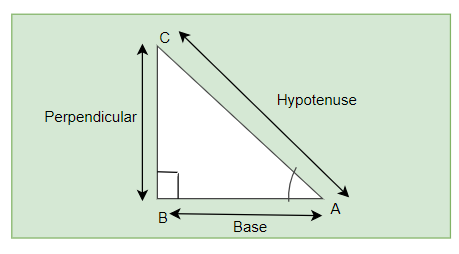• We can define following term for angle A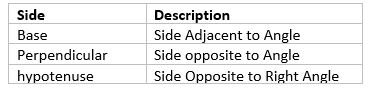• We can define the trigonometric ratios for angle A as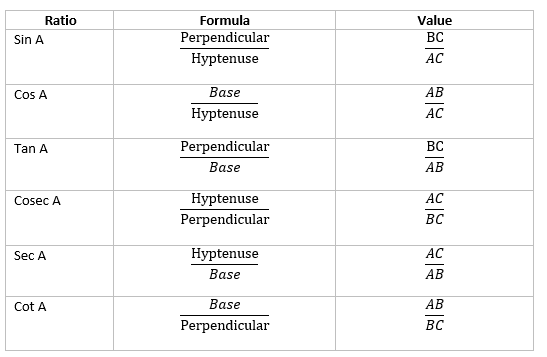Notice that each ratio in the right-hand column is the inverse, or the reciprocal, of the ratio in the left-hand column.
The reciprocal of sin A is cosec A ; and vice-versa.
The reciprocal of cos A is sec A
And the reciprocal of tan A is cot A
These are valid for acute angles.
• We can define $\tan A = \frac {\sin A}{\cos A}$
And $Cot A =\frac {cos A}{ Sin A}$

Important Note
Since the hypotenuse is the longest side in a right triangle, the value of
sin A or cos A is always less than 1 (or, in particular, equal to 1).
• Similarly we can have define these for angle $C$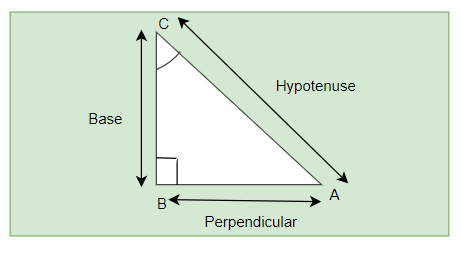We can define the trigonometric ratios for angle $C$  as
$\sin C= \frac {\text{Perpendicular}}{\text{Hypotenuse}}=\frac {AB}{AC}$
$\csc C= \frac {\text{Hypotenuse}}{\text{Perpendicular}} =\frac {AC}{AB}$
$\cos C= \frac {\text{Base}}{\text{Hypotenuse}}=\frac {BC}{AC}$
$\sec C= \frac {\text{Hypotenuse}}{\text{Base}}=\frac {AC}{BC}$
$\tan C= \frac {\text{Perpendicular}}{\text{Base}}=\frac {AB}{BC}$
$\cot C= \frac {\text{Base}}{Perpendicular}=\frac {BC}{AB}$

## How to learn this trigonometric ratio's

Two ways

English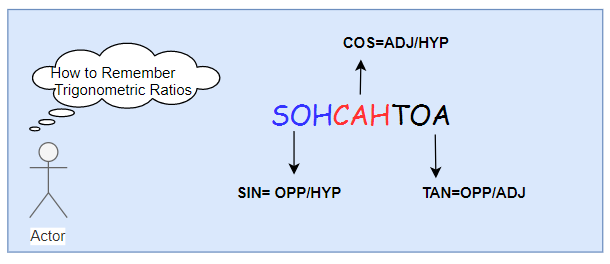Hindi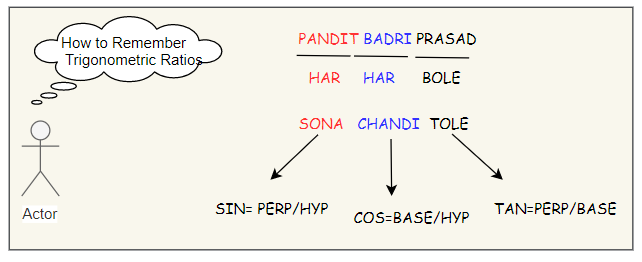## Trigonometric Ratios of Common angles

We can find the values of trigonometric ratio’s various angle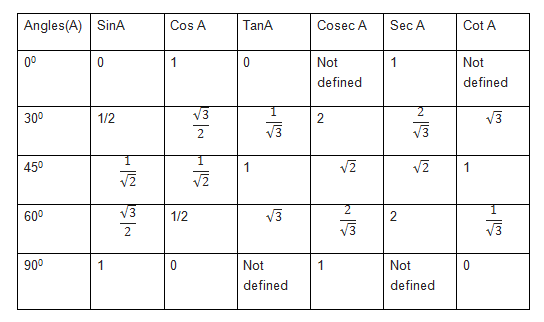Important things
• From the table we can see that cosec 0 is undefined. It is undefined as cosec A= hyp/Perp,when angle becomes zero, Perpendicular becomes zero and x/0 is undefined
• Similarly for Cot 0
• From the table we can see that ,tan 90 is undefined . It is undefined as tan A=perp/base ,when angle becomes 90,base becomes zero and x/0 is undefined
• Similarly , it can be explained for sec 90
Question 1
(i) The value of $\tan A$ is always less than 1.
(ii) $\sec A =\frac {12}{5}$ for some value of angle A.
(iii) cos A is the abbreviation used for the cosecant of angle A.
(iv) tan A is the product of tan and A.
(v) $\sin A =\frac {5}{3}$ for some angle
Solution
(i) False . The value of tan A increase from 0 to infinity
(ii) True . The value of sec A increase from 1 to infinity
(iii) False .Cos A is the abbreviation used for the cosine of angle A
(iv) False .cot A is one symbol. We cannot separate it
(v) False. The value of sin AA always lies between 0 and 1 and 5/3 > 1
Question 2
The value of $(\sin 30 + \cos 30) - (\sin 60 + \cos 60)$ is
(A) - 1
(B) 0
(C) 1
(D) 2
Solution

## Trigonometric ratio’s of complimentary angles

We know that for Angle A, the complementary angle is 90 – A
In a right angle triangle ABC
$A+B+C=180$
Now $B=90$
So $A +C =90$
Or $C=90-A$

We have seen in Previous section the value for  trigonometric ratios for angle $C$

$\sin C= \frac {\text{Perpendicular}}{\text{Hypotenuse}}=\frac {AB}{AC}$
$\csc C= \frac {\text{Hypotenuse}}{\text{Perpendicular}} =\frac {AC}{AB}$
$\cos C= \frac {\text{Base}}{\text{Hypotenuse}}=\frac {BC}{AC}$
$\sec C= \frac {\text{Hypotenuse}}{\text{Base}}=\frac {AC}{BC}$
$\tan C= \frac {\text{Perpendicular}}{\text{Base}}=\frac {AB}{BC}$
$\cot C= \frac {\text{Base}}{\text{Perpendicular}}=\frac {BC}{AB}$

This can be rewritten as

$\sin (90^{\circ}-A) =\frac {AB}{AC}$
$\csc (90^{\circ}-A) =\frac {AC}{AB}$
$\cos (90^{\circ}-A) =\frac {BC}{AC}$
$\sec (90^{\circ}-A)=\frac {AC}{BC}$
$\tan (90^{\circ}-A)=\frac {AB}{BC}$
$\cot( 90^{\circ}-A) =\frac {BC}{AB}$

Also we know that

$\sin A= \frac {BC}{AC}$
$\csc A= \frac {AC}{BC}$
$\cos A= \frac {AB}{AC}$
$\sec A = \frac {AC}{AB}$
$\tan A= \frac {BC}{AB}$
$\cot A =\frac {AB}{BC}$

From both of these, we can easily make it out
$\sin (90^{\circ}-A) =\cos A$
$\cos(90^{\circ}-A) = \sin A$
$\tan(90^{\circ}-A) =\cot A$
$\sec(90^{\circ}-A)= \csc A$
$\csc (90^{\circ}-A) =\sec A$
$\cot(90^{\circ}- A) =\tan A$

## Trigonometric identities

We have studied Pythagoras theorem in earliar classes which states that for a right-angled triangle the square on the hypotenuse is equal to the sum of the squares on the other two side
If a is the hypotenuse and b and c are other two sides,then
$a^2= b^2+ c^2$

This same theorem can be used to prove the below trigonometric identities
$\sin^2 A + \cos^2 A =1$
$1 + \tan^2 A =\sec^2 A$
$1 + \cot^2 A =\csc^2 A$

## How to solve Trigonometric Identities Problems

1. Learn well the formulas for Trigonometric identities, trigonometric ratios, reciprocals The better you know the basic identities, the easier it will be to recognize what is going on in the problems.
2. Generally RHS( Right hand side) would be more complex. So start from there and simplify it to the same form as LHS(Left hand side)
3. It becomes many times easy, if Convert all sec, csc, cot, and tan to sin and cos. Most of this can be done using the quotient and reciprocal identities.
4. If you see power 2 or more, it will involve using the below identities mostly
$\sin^2 A + \cos^2 A =1$
$1 + \tan^2 A =\sec^2 A$
$1 + \cot^2 A =\csc^2 A$
5. Once you get the hang of it, you will begin to see patterns and it will be easy to solve these Trigonometric identities Problems
6. Practice and Practice. You will soon start figuring out the equation and there symmetry to resolve them fast
Question 1
Prove that $(\sin^4 \theta - \cos^4 \theta +1) \csc^2 \theta = 2$
Solution
L.H.S. = $(\sin^4 \theta - \cos^4 \theta +1) \csc^2 \theta$
$= [(\sin^2 \theta - \cos^2 \theta) (\sin^2 \theta + \cos^2 \theta) + 1] \csc^2 \theta$
$= (\sin^2 \theta - \cos^2 \theta) + 1) \csc^2 \theta$
[Because $\sin^2 A + \cos^2 A =1$]
$= 2 \sin^2 \theta \csc^2 \theta$ [Because $1- \cos^2 \theta = \sin^2 \theta$ ]
= 2 = RHS
Crossword Puzzle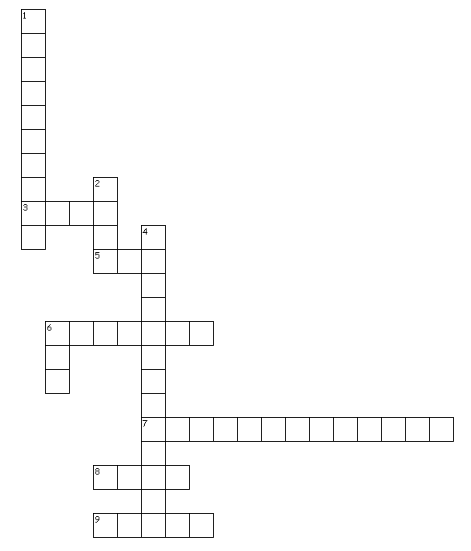Across
3. Reciprocal of the trigonometric ratio cosecant
5. Value of cosine of 0 degrees
6. Ratio obtained by dividing sine by cosine
7. Two angles whose sum is 90 degrees
8. Value of sine of 30 degrees
9. The value of $(1+ 2 \sin 90^0) (1- \sin 0^0. \sin 10^0. \sin 30^0. \sin 80^0. \sin 90^0)$
Down
1. Ratio of side adjacent to angle and cosine
2. Value of cosine of 90 degrees
4. side opposite to angle
6. Value of cosecant 30 degrees

### Quiz Time

Question 1 Which one of them is true ?
A) $sin A = sin \times A$
B) $sin 90= 2 sin 45$
C) $cos 75= cos 30 + cos 45$
D) $tan A= \frac {sinA}{cosA}$
Question 2 Which one of them is false ?
A) sin30 =1/2
B) cos 0=1
C) cos 45=1/2
D) sin 0=0
Question 3 Which is of these is a trigonometric identity
A) $sin A + cos A = 1$
B) $1 + cot^2 A =cosec^2 A$
C) $sin^2 A - cos^2 A = 1$
D) None of these
Question 4 What is the value of $Sin60^0 cos30^0+sin30^0 cos60^0$
A) 1
B) 2
C) 0
D) -1
Question 5 sin(90 -A) =?
A) Sin A
B) tan A
C)cos A
D) cosec A
Question 6 What is the value of $\sqrt {1+ tan^2A}$ where A is acute angle ?
A) cot A
B) sin A
C)cos A
D) sec A

## Summary

Here is the summary for class 10 trigonometry notes
• In a right angle triangle, where $\angle B =90^0$, we have
$sin A =\frac {\text{side Opposite to A}}{\text{hypotenuse}}$
$cos A =\frac {\text{side Adjacent to A}}{\text{hypotenuse}}$
$tan A =\frac {\text{side Opposite to A}}{\text{side Adjacent to A}}$
The reciprocal of sin A is cosec A ; and vice-versa.
The reciprocal of cos A is sec A
And the reciprocal of tan A is cot A
• $sin A \le 1$ ,$cos A \le 1$ , $sec A \ge 1$,$cosec A \ge 1$
• Following are the trigonometric identities $\sin^2 A + \cos^2 A =1$
$1 + \tan^2 A =\sec^2 A$
$1 + \cot^2 A =\csc^2 A$Go back to Class 10 Main Page using below links

### Practice Question

Question 1 What is $1 - \sqrt {3}$ ?
A) Non terminating repeating
B) Non terminating non repeating
C) Terminating
D) None of the above
Question 2 The volume of the largest right circular cone that can be cut out from a cube of edge 4.2 cm is?
A) 19.4 cm3
B) 12 cm3
C) 78.6 cm3
D) 58.2 cm3
Question 3 The sum of the first three terms of an AP is 33. If the product of the first and the third term exceeds the second term by 29, the AP is ?
A) 2 ,21,11
B) 1,10,19
C) -1 ,8,17
D) 2 ,11,20